Mathematical and Physical Journal
for High Schools
Issued by the MATFUND Foundation
 Already signed up? New to KöMaL?

# Exercises and problems in PhysicsDecember 2003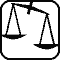## Experimental problem

M. 247. Connect the bulb of an electric torch of a given nominal power to a 4,5 V battery. Measure how the actual power of the bulb changes after the connection during a long time. Determine the energy picked up by the bulb as a function of time.## Theoretical problems

It is allowed to send solutions for any number of problems, but final scores of students of grades 9-12 are computed from the 5 best score in each month. Final scores of students of grades 1-8 are computed from the 3 best scores in each month.

P. 3662. When a jam-filled sweet roll is heated in a microwave oven, the jam gets hotter than the pastry. Why?

(3 points)

P. 3663. Two cats are running along two perpendicular streets of a town built on a plane. The mass of one (Culu) is 2 kg and its speed is 4 m/s. The mass of the other (Mulu) is 6 kg and its speed is 2 m/s. a) What is the speed of Culu compared to Mulu? b) Which cat has the greater velocity in their common mass centre system, and how many times is it greater? c) In which frame of reference is their kinetic energy at minimum? What is this value?

(4 points)

P. 3664. The aerodynamic drag exerted on a car driving at a speed of 72 km/h is three times higher in head-wind than in back-wind. How many times is the aerodynamic drag higher in head-wind than in no wind?

(4 points)

P. 3665. We make a circular immersion heater of a radius of 3 cm of a thin heater coil, and then we dip it into water. How long does it take for this heater connected to the 230 V electric network to heat up 0.5 of water from 10 oC to 60 oC, if the maximum of the generated magnetic induction in the centre of the circle is 2.10-5 T and the efficiency of the heating is 80%?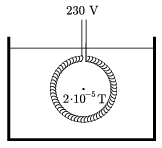(4 points)

P. 3666. There are two ways to get some helium gas from state A to B (see figure). In which case will the ratio of the work done to the heat absorbed be greater?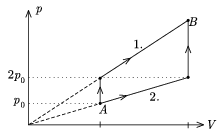(5 points)

P. 3667. Two parallel rails running in a b distance from each other are connected through a capacitor of capacity C. In $\displaystyle \ell$ distance from the capacitor a metal wire of mass m is laid perpendicularly across the rails. The resultant frame is then put into a homogeneous magnetic field so that the plane of the frame includes an angle $\displaystyle \alpha$ with the horizontal plane. The direction of the B magnetic induction vector is vertical. Friction and ohmic resistance is negligible.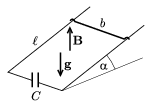At what speed does the wire reach the end of the slope?

(5 points)

P. 3668. In the ideal oscillating circuit shown in the figure the capacity of the capacitor is 25 $\displaystyle \mu$F, the voltage is 10 V and the inductance of the coil is 0.04 H. What will the highest value of the electric current be in the oscillating circuit after closing switch K?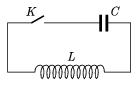(4 points)

P. 3669. The mass of the astronauts in a space station cannot be checked with the scales used on the Earth. Imagine a seat of a mass of 20 kg which, in an elastic suspension, oscillates with a frequency of 2 Hz. After the astronaut sits in the seat the frequency decreases to 1 Hz. How much is the mass of the astronaut?

(4 points)

P. 3670. On a tabletop of some friction a table-tennis ball rolls clearly toward a perfectly smooth wall perpendicularly to the plane of the wall. Can it happen that the ball collides with the wall exactly two times? (Collisions happen in no time and they are not perfectly elastic.)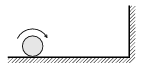(5 points)

P. 3671. The cogs of a glass cog-wheel are optical prisms of congruent isosceles triangle shaped cross sections. Their interesting property is that they can lead the light around'' the cog-wheel. What is the angle of refraction of the prisms if they fit on the sides of a regular polygon of N sides. The refractive index of the glass is n=1.5. Let, for example, N=12. Examine the case when N=3, too.

(5 points)